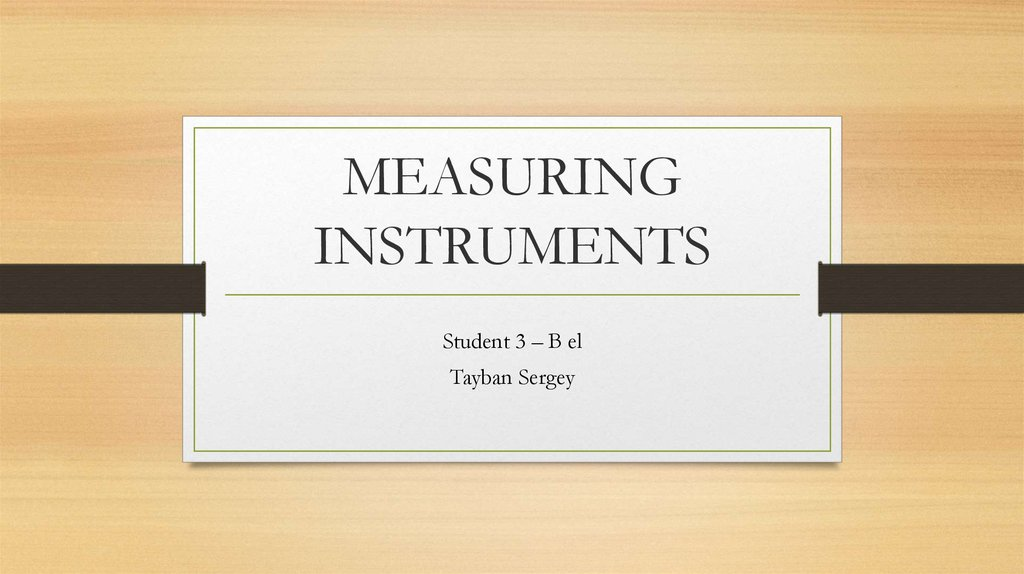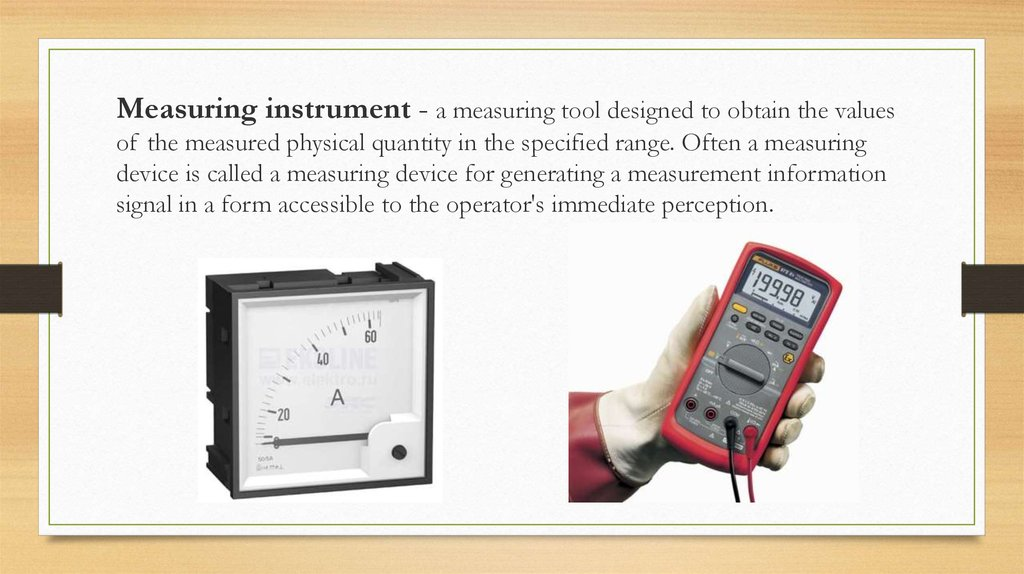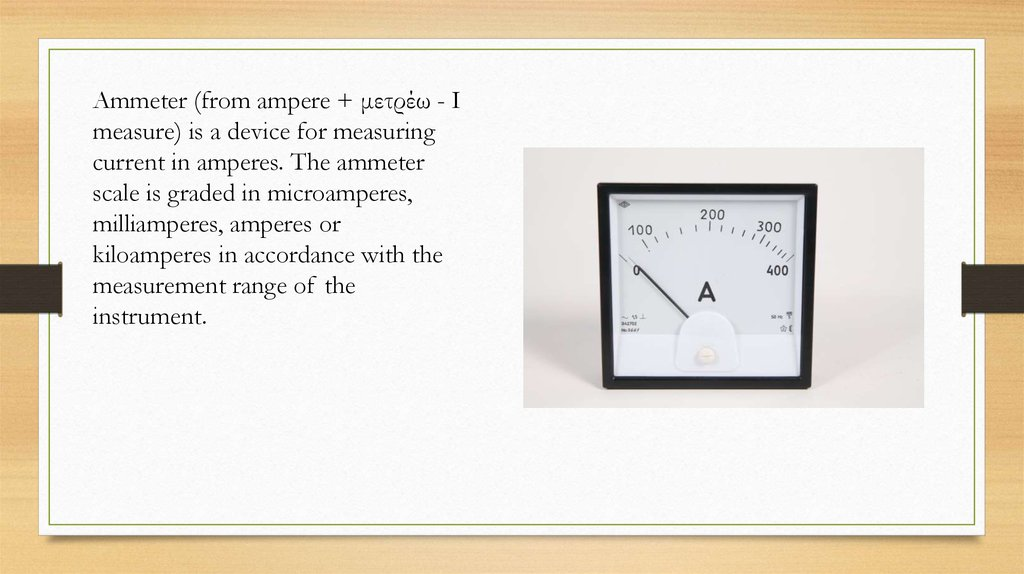# Measuring instruments

Student 3 – B el
Tayban Sergey

## 2. Measuring instrument - a measuring tool designed to obtain the values of the measured physical quantity in the specified range. Often a measuring device is called a measuring device for generating a measurement information signal in a form accessible to t

Measuring instrument - a measuring tool designed to obtain the values
of the measured physical quantity in the specified range. Often a measuring
device is called a measuring device for generating a measurement information
signal in a form accessible to the operator's immediate perception.

## 3.

Ammeter (from ampere + μετρέω - I
measure) is a device for measuring
current in amperes. The ammeter
milliamperes, amperes or
kiloamperes in accordance with the
measurement range of the
instrument.

## 4.

In the electrical circuit, the ammeter is
connected in series with that portion of the
electrical circuit whose current strength is
measured. Therefore, the lower the internal
resistance of the ammeter (ideally - 0), the
less will the effect of the instrument on the
object under study, and the higher the
accuracy of the measurement. To increase the
measurement limit, the ammeter is supplied
with a shunt (for AC and DC circuits), a
current transformer (only for AC circuits), or
a magnetic amplifier (for DC circuits). It is
very dangerous to try to use an ammeter as a
voltmeter (connect it directly to a power
source): this can lead to short circuits!

## 5.

The voltmeter (volts + gr. Μετρεω
measure) is a direct reading instrument
for determining the voltage or EMF in
electrical circuits. It is connected in
parallel to the load or the source of
electrical energy.

## 6.

An ideal voltmeter should have an
infinitely large internal resistance.
Therefore, the higher the internal
resistance in a real voltmeter, the
less influence the device has on
the measured object and,
consequently, the higher the
accuracy and the more diverse the
field of application. Voltmeters are
connected to the network in
parallel.

## 7.

The ohmmeter (Ohm + other-Greek
μετρεω "measure") is a direct reading
device for determining electrical
resistive (ohmic) resistances. Normally,
the measurement is done by direct
current, however, in some electronic
ohmmeters, alternating current can be
used. Varieties of ohmmeters: megaohmmeters, giga-meters,
teramometers, milliammeters, micrometers, differing in the ranges of
measured resistances.

## 8.

Electricity meter (electric meter) is a device for measuring electricity
consumption of alternating or direct current (usually in kWh or Ah).

Student 3 – B el
Tayban Sergey